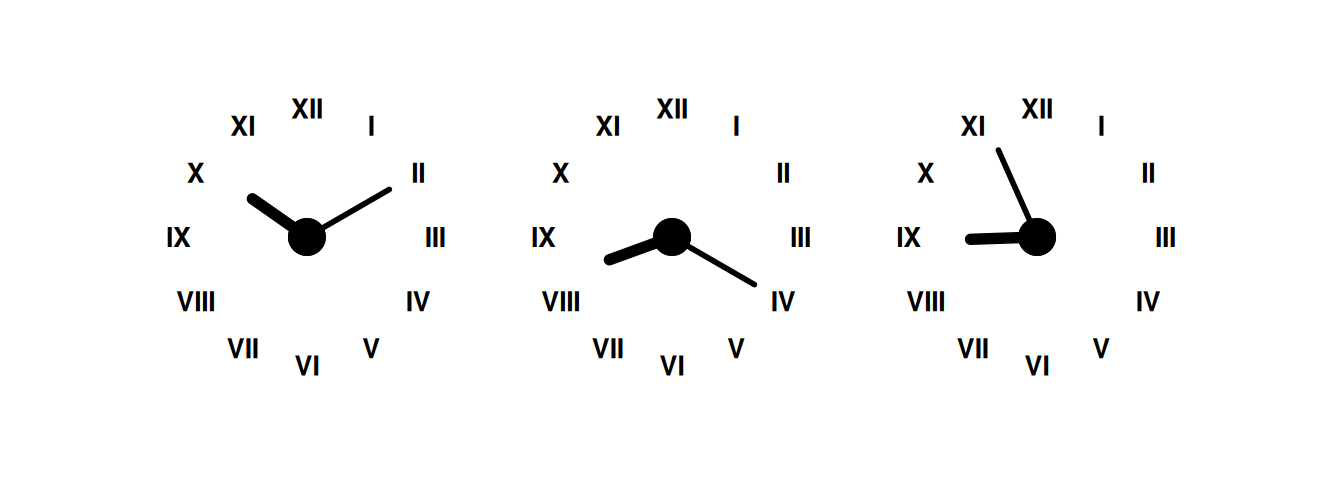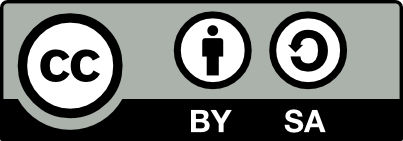## 11.59 Text Path Clock Multiple Plots

20220109clock <- function(x)
{
hours <- c(rep(x %% 12 + tail(x, 1) / 60, 2), 0, 3.5)
minutes <- c(rep(tail(x, 1)/5, 2), 0, 5)

as.data.frame(rbind(hours, minutes)) %>%
ggplot(aes(V1, V3)) +
geom_segment(aes(xend=V2, yend=V4),
size=c(2, 1),
lineend="round") +
geom_point(x=0, y=0, size=6) +
scale_x_continuous(limits=c(0, 12),
breaks=1:12,
label=as.roman) +
scale_y_continuous(limits=c(0, 6),
expand=c(0, 0)) +
theme_void() +
theme(plot.margin=unit(c(30,15,30,15), "pt"),
axis.text.x=element_text(size=10, face=2))
}

c1 <- clock(10:10) + coord_polar()
c2 <- clock(8:20) + coord_polar()
n <- now(); h <- lubridate::hour(n); m <- lubridate::minute(n)
c3 <- clock(h:m) + coord_polar()

c1 + c2 + c3

The clock is an example from geomtextpath and demonstrates a nice hack to produce a clock face. Any time can be specified in the call to clock().

In our example we have three clocks generated and using patchwork the multiple plots are displayed as a single plot through the + operator.

### References

Cameron, Allan, and Teun van den Brand. 2022. Geomtextpath: Curved Text in Ggplot2. https://allancameron.github.io/geomtextpath/.
Pedersen, Thomas Lin. 2022. Patchwork: The Composer of Plots. https://CRAN.R-project.org/package=patchwork.

Your donation will support ongoing availability and give you access to the PDF version of this book. Desktop Survival Guides include Data Science, GNU/Linux, and MLHub. Books available on Amazon include Data Mining with Rattle and Essentials of Data Science. Popular open source software includes rattle, wajig, and mlhub. Hosted by Togaware, a pioneer of free and open source software since 1984. Copyright © 1995-2022 Graham.Williams@togaware.com Creative Commons Attribution-ShareAlike 4.0